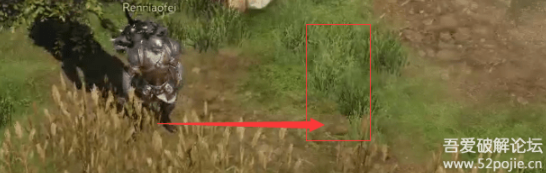2022-9-24 08:3:58 Author: Web安全工具库(查看原文) 阅读量:14 收藏

1.游戏为什么会产生瞬移?

2.那么新游戏存在不存在瞬移呢?

3.什么是加速?

4.了解了这些,我们就来看看, LOSTARK 是怎么瞬移加速的吧

LOSTARK调试的建议(该部分不需要可以不看)

(不是轻微的卡顿，是完全没有办法控制)

``朝向 = *(DWORD*)(*(QWORD*)(对象2 + 0x90) + 0x4B4);``

4B4的偏移 我们没有看到也算正常。

X正朝向为0 和FFFF(朝向必有一个朝向是最大值和最小值重叠的)
Y正朝向为 3FFF
X负朝向 7FFE
Y负朝向 BFFD

``void Call_瞬移(FLOAT X, FLOAT Y, FLOAT Z)``{``extern QWORD 角色基地址2;``QWORD Temp = *(QWORD*)角色基地址2;``Temp = *(QWORD*)(Temp + 0x440);``QWORD 对象2 = *(QWORD*)(Temp + 0x18);`` ``*(FLOAT*)(*(QWORD*)(对象2 + 0x90) + 0x7C) = X;``*(FLOAT*)(*(QWORD*)(对象2 + 0x90) + 0x80) = Y;``*(FLOAT*)(*(QWORD*)(对象2 + 0x90) + 0x84) = Z;``}``void CTestDialog::OnBnClickedButton19()``{``UpdateData(TRUE);``Call_瞬移(m_edit3, m_edit4, m_edit5);``}``

(如果我们连续短距离瞬移，中间不走路来让服务器确认我们的坐标有效性和直接长距离瞬移是没有区别的，还是会被修正回来的)

``if (A.朝向 > 0 && A.朝向 < 0x3FFF)``{``X增 = (FLOAT)(200 * sin((0x3FFF - A.朝向) * 2 * 3.14159 / 0xFFFF));``Y增 = (FLOAT)(200 * cos((0x3FFF - A.朝向) * 2 * 3.14159 / 0xFFFF));``Call_瞬移(A.X + X增, A.Y + Y增, A.Z);``}``

``if (A.朝向 > 0xFFFF * 3 / 4 && A.朝向 < 0xFFFF)``{``X增 = (FLOAT)(200 * sin((A.朝向 - 0xFFFF * 3 / 4) * 2 * 3.14159 / 0xFFFF));``Y增 = (FLOAT)(200 * cos((A.朝向 - 0xFFFF * 3 / 4) * 2 * 3.14159 / 0xFFFF));``Call_瞬移(A.X + X增, A.Y - Y增, A.Z);``}``

``if (A.朝向 > 0xFFFF / 2 && A.朝向 < 0xFFFF * 3 / 4)``{``X增 = (FLOAT)(200 * sin((0xFFFF * 3 / 4 - A.朝向) * 2 * 3.14159 / 0xFFFF));``Y增 = (FLOAT)(200 * cos((0xFFFF * 3 / 4 - A.朝向) * 2 * 3.14159 / 0xFFFF));``Call_瞬移(A.X - X增, A.Y - Y增, A.Z);``}``

``if (A.朝向 > 0xFFFF / 2 && A.朝向 < 0xFFFF * 3 / 4)``{``X增 = (FLOAT)(200 * sin((0xFFFF * 3 / 4 - A.朝向) * 2 * 3.14159 / 0xFFFF));``Y增 = (FLOAT)(200 * cos((0xFFFF * 3 / 4 - A.朝向) * 2 * 3.14159 / 0xFFFF));``Call_瞬移(A.X - X增, A.Y - Y增, A.Z);``}``

``void  Call_向前瞬移200()``{``人物属性 A;``A.初始化();``FLOAT X增;``FLOAT Y增;``  ``if (A.朝向 > 0 && A.朝向 < 0x3FFF)``{      ``X增 = (FLOAT)(200 * sin((0x3FFF - A.朝向) * 2 * 3.14159 / 0xFFFF));``Y增 = (FLOAT)(200 * cos((0x3FFF - A.朝向) * 2 * 3.14159 / 0xFFFF));``Call_瞬移(A.X + X增, A.Y + Y增, A.Z);``}``if (A.朝向 > 0xFFFF * 3 / 4 && A.朝向 < 0xFFFF)``{``X增 = (FLOAT)(200 * sin((A.朝向 - 0xFFFF * 3 / 4) * 2 * 3.14159 / 0xFFFF));``Y增 = (FLOAT)(200 * cos((A.朝向 - 0xFFFF * 3 / 4) * 2 * 3.14159 / 0xFFFF));``Call_瞬移(A.X + X增, A.Y - Y增, A.Z);``}``if (A.朝向 > 0xFFFF / 2 && A.朝向 < 0xFFFF * 3 / 4)``{``X增 = (FLOAT)(200 * sin((0xFFFF * 3 / 4 - A.朝向) * 2 * 3.14159 / 0xFFFF));``Y增 = (FLOAT)(200 * cos((0xFFFF * 3 / 4 - A.朝向) * 2 * 3.14159 / 0xFFFF));``Call_瞬移(A.X - X增, A.Y - Y增, A.Z);``}``if (A.朝向 > 0x3FFF && A.朝向 < 0xFFFF / 2)``{``X增 = (FLOAT)(200 * sin((A.朝向 - 0x3FFF) * 2 * 3.14159 / 0xFFFF));``Y增 = (FLOAT)(200 * cos((A.朝向 - 0x3FFF) * 2 * 3.14159 / 0xFFFF));``Call_瞬移(A.X - X增, A.Y + Y增, A.Z);``}`` ``}``

``void Call_凌波微步(FLOAT X, FLOAT Y, FLOAT Z)``{`` ``人物属性 A;``A.初始化();``int i = 0;`` ``FLOAT 距离 = sqrt((A.X - X)*(A.X - X) + (A.Y - Y)*(A.Y - Y) + (A.Z - Z)*(A.Z - Z));``while (距离>50)``{``i++;``if (i > 100) break;`` ``Call_向前瞬移200();``        Sleep(80);``Call_寻路(X, Y, Z);``Sleep(120);``A.初始化();``距离 = sqrt((A.X - X)*(A.X - X) + (A.Y - Y)*(A.Y - Y) + (A.Z - Z)*(A.Z - Z));``}``}``

1.检测无需实时, 抽样调查,减少服务器压力, 而且每人又会不知什么情况下被检测

2.做单点检测 不可能完美,  做区间测速

3.玩家举报
4.相关原理,过程代码的检测